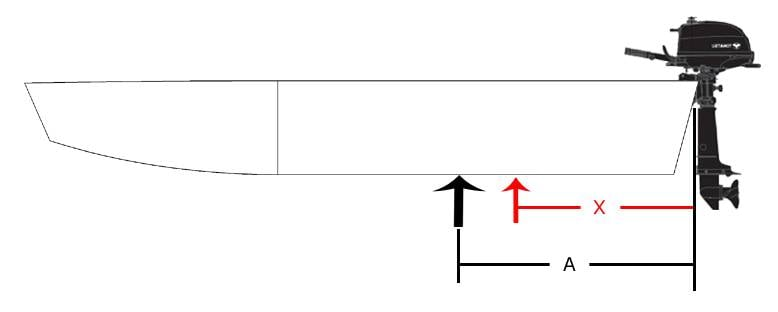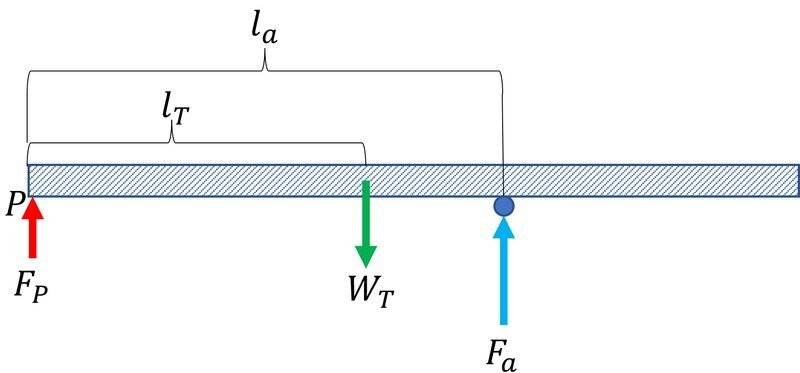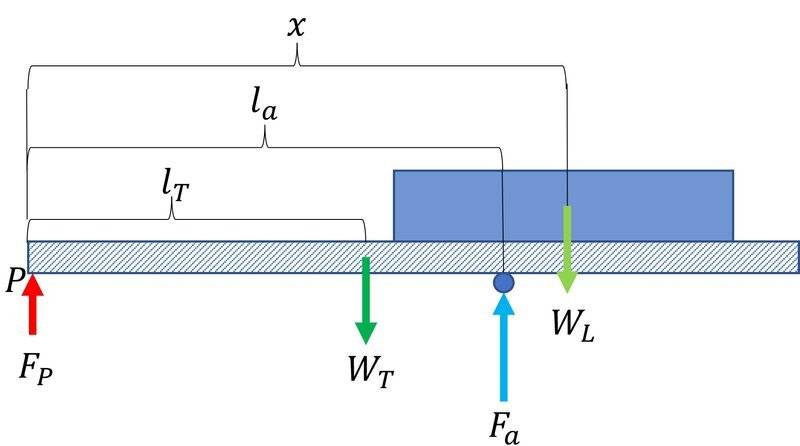# Proper placement of boat on trailer

• I
HRG
New trailer and new self built boat. Need confirmation of my calculation for placement of boat on trailer to have the correct tongue weight.

For those not familiar with trailers:
1. The tongue of a trailer is the forward beam that has the female ball receiver at the front end.
2. It is imperative that the weight of the trailer at the ball be 10-15 % of the total weight of the "trailer, boat, motor and anything in the boat". This results in steady, sway free pulling of the trailer by the vehicle at highway speeds.
3. The commercially sold trailer with nothing on it will have the proper tongue weight for the empty trailer, to allow pulling the empty trailer safely. In other words, if the weight of the trailer is 300 lbs, then the tongue weight might be 10% or 30 lbs.
Here's a picture of my boat:Assume that the total weight of the boat and motor is 250 lbs. When the boat is placed on the trailer, the total weight of trailer + boat will be 550 lbs (300lbs + 250lbs).

The black arrow points to the balance point of the boat with motor. If the boat is placed on the trailer with the black arrow over the wheel's axle, then the tongue weight will remain as 30 lbs which is wrong. The tongue weight at 10% of the total weight needs to be 55 lbs.

The red arrow points to where the boat should be placed over the axle to get 55 lbs of tongue weight.

My calculation is that X should be 80% of A to have the forward portion of the boat from the red arrow be 10% heavier than the aft portion of the boat from the red arrow. In other words, X=0.8A. Then if the boat is placed on the trailer with the red arrow above the axle, the tongue weight will be 10% of the total weight of the trailer + boat.

Is my calculation correct?

Gold Member
When the boat is placed on the trailer, the total weight of trailer + boat will be 550 lbs (300lbs + 250lbs).
250 lbs of boat load to trailer is same for red X and black A in the figure, it seems. 300/550=54.5% is too much for the regulation.

•pbuk
Staff Emeritus
Homework Helper
Gold Member
My calculation is that X should be 80% of A to have the forward portion of the boat from the red arrow be 10% heavier than the aft portion of the boat from the red arrow. In other words, X=0.8A. Then if the boat is placed on the trailer with the red arrow above the axle, the tongue weight will be 10% of the total weight of the trailer + boat.

Is my calculation correct?
How could we possibly answer that if you do not show us your calculation and assumptions?

It is also not a question of which part of the boat goes over the wheel’s axle. It is a question of placing the boat’s center of mass at the same position as the trailer’s center of mass. The question therefore seems fundamentally misguided.

HRG
anuttarasammyak and Orodruin,

... How could we possibly answer that if you do not show us your calculation and assumptions? ...

I thought that folks would do the calculation using their own method and let me know if the result agreed with mine.

Here's my assumption and calculation:

Assume there is a 4" diameter round rod under the boat at the black arrow. The boat balances on the rod. There is zero weight held up by the trailer hitch ball.

Now if the rod is placed under the transom of the boat, and assuming that the weight of the motor is above that, 50% of the weight of the boat will be held up by the ball of the trailer hitch.

Here is a diagram of the boat in character format:
Code:
==================================
@                  ^   !         O

Where:
@ is the trailer hitch ball
^ is the balance point (black arrow)
! is the red arrow
O is the round rod under the transom.

So 50% of the boat's weight is being held up by the trailer hitch ball when the round rod is under the transom. In other words, moving the round rod from the balance point (^) to under the transom (O) increases the weight on the trailer hitch ball by 50% of the weight of the boat.

Using the distance from ^ to O as 100% of the movement of the rod, the weight on the trailer hitch ball increases from zero to 50%. In percentage groups, the distance from ^ to O goes 20%, 40%, 60%, 80% 100% = 5 groups. Each group = 10% increase in the tongue weight at the hitch ball. (5 groups at 10% each = 50% weight increase)

So group-1, the 20% = 10% weight increase on the hitch ball.

The balance "X" = 80%.
That is how I came up with X=0.8A
Crude calculation but maybe close enough to install the two supports (bunks) to hold up the boat on the trailer.

Last edited:
Staff Emeritus
Homework Helper
Gold Member
Now if the rod is placed under the transom of the boat, and assuming that the weight of the motor is above that, 50% of the weight of the boat will be held up by the ball of the trailer hitch.
Why? This does not seem correct to me. As I said already, you cannot possibly hope to solve your problem like this. What you need to consider is torque equilibrium around the wheel axis. It is not about placing a certain percentage of the boat weight on either side of the axis. It is about ensuring that the lever arm of the boat center of mass is 10-15% of the distance to the ball.

Depending on the size of the boat and the size of the trailer, this can mean anything from putting the entire boat in front of the wheel axis to almost equal amounts of boat on either side.

HRG
Why? This does not seem correct to me. As I said already, you cannot possibly hope to solve your problem like this. What you need to consider is torque equilibrium around the wheel axis.
The tongue weight is established statically, not dynamically. For a small trailer and boat like mine, one method is to have the trailer level and support the hitch ball receiver on a bathroom scale. The static weight needs to be 10-15% of the total weight of trailer, boat and contents.

Not sure how "torque equilibrium around the wheel axis" (that sounds like a dynamic measurement) would affect the tongue weight.

Thanks for the help.

Staff Emeritus
Homework Helper
Gold Member
The tongue weight is established statically, not dynamically.
Yes? So? You still need to consider torque equilibrium to find out how the weight is distributed between axle and ball.

that sounds like a dynamic measurement
It is not.

Of course, you could just do this experimentally by weighing but you asked how to do the computation.

Homework Helper
2022 Award
If the unladen trailer has a 15% tongue load then its center of mass is 15% of the distance from wheel to ball socket. Mark that spot on the trailer. Put the center of mass of the boat on that spot. Check with bath scale. Done

•erobz and pbuk
Staff Emeritus
Homework Helper
Gold Member
If the unladen trailer has a 15% tongue load then its center of mass is 15% of the distance from wheel to ball socket. Mark that spot on the trailer. Put the center of mass of the boat on that spot. Check with bath scale. Done
As stated:
It is about ensuring that the lever arm of the boat center of mass is 10-15% of the distance to the ball.

This is what provides torque equilibrium at the wheel axle with the required tongue load.

My point was that if you want to compute it, it will not be a matter of making the red distance x in the OP a certain percentage of the black distance A.

Homework Helper
2022 Award
-Sorry my comment was not really directed at your response and I agree with what you said. My attempt was to start afresh to show that the OP was complicating the matter unnecessarilly.

Homework Helper
Gold Member
If the unladen trailer has a 15% tongue load then its center of mass is 15% of the distance from wheel to ball socket. Mark that spot on the trailer. Put the center of mass of the boat on that spot. Check with bath scale. Done
Yes, this is exactly what to do, although you can calculate this spot too.

Last edited:
Homework Helper
Gold Member
Is my calculation correct?
No because it doesn't take account of a key piece of information: the distance from the axle to the towing hitch.

If we assume the unladen trailer has a balanced hitch we can ignore its weight. We then simply need to put the centre of mass of the trailer at the point where 10-15% of its weight is on the hitch. We can do this by putting the COM 10-15% of the distance from the axle to the hitch. You will find that the point mentioned by @hutchphd is in this range (assuming the trailer has a 10-15% hitch weight).

•hutchphd
Gold Member
I would like to correct my post #2. For a simplified model equation of zero torque is
$$W_t x =(W-W_t)(L-x)$$
So
$$W_t = \frac{W(L-x)}{L}$$
where
##W_t##: tongue weight
W : sum of weights of towed trailer and cargo load
L : horizontal distance between tongue and Earth contact points of trailer
x : horizontal distance of COM of total weight W measured from the tongue

Video in
https://www.legendboats.com/?blog=tongue-weight-the-key-to-safe-towing#:~:text=Tongue weight is the downwards,rear axle of your vehicle.
was informative.

Last edited:
••Lnewqban and pbuk
Homework Helper
Gold Member
$$W_t = \frac{W(L-x)}{L}$$
Rearranging this so it is more useful for the OP: $$\frac{(L-x)}{L} = \frac{W_t}{W}$$, which in words is "if you want X% of the weight on the hitch then put the centre of mass X% forward of the axle", which is what I said in #12.

Gold Member
Disclaimer: The diagrams are not meant to represent how the trailer should be loaded. They are only meant to serve as a reference for the quantities described in the supporting analysis.From a force balance we have that:

$$F_P = f W_T$$

$$F_a = \left( 1-f \right) W_T$$

Where ## f## is the design fraction of the trailer ( trailer + load ) weight supported by the tongue, and ##F_a## is the reaction force at the axle:

If we sum torques about ##P##

$$\circlearrowright ^+ \sum \tau = l_T W_T - l_a \left( 1-f\right)W_T = 0 \implies l_T W_T = l_a \left( 1-f\right)W_T \tag{1}$$

Performing the same analysis for a loaded trailer:$$F_P = f \left( W_T + W_L \right)$$

$$F_a = \left( 1-f\right)\left( W_T + W_L \right)$$

$$\circlearrowright ^+ \sum \tau = l_a \left( 1- f \right) \left( W_T+W_L\right) - l_T W_T - x W_L = 0 \tag{2}$$

where ## x ## is the horizontal distance from ##P## to the load center of mass.

We can then substitute our result for ## l_TW_T## from ##(1) \to (2) ##:

$$l_a \left( 1- f \right) \left( W_T+W_L\right) -l_a \left( 1-f\right)W_T - x W_L = 0$$

And after simplification, the distance to the load center of mass ##x## required to preserve the design fraction of total load ##f## supported at ##P## is given by:

$$\boxed{ x = l_a \left( 1-f \right) }$$

For this problem the OP has referenced ##f## as:

$$0.10 < f < 0.15$$

Last edited:
•Lnewqban
Homework Helper
Gold Member
https://www.physicsforums.com/attachments/304346
This diagram has the CoM of the load behind the axle: that is not a good idea. Your workings are also complicated by the fact that you are using the subscript T to mean both 'tow hitch' (as in ## f_T ## and 'trailer' (as in ## W_T ##). The answer is the same as already posted in #14 and #12 which I will quote again here:
$$\frac{(L-x)}{L} = \frac{W_t}{W}$$ which in words is "if you want X% of the weight on the hitch then put the centre of mass X% [of the distance of the hitch] forward of the axle".

Gold Member
This diagram has the CoM of the load behind the axle: that is not a good idea. Your workings are also complicated by the fact that you are using the subscript T to mean both 'tow hitch' (as in ## f_T ## and 'trailer' (as in ## W_T ##). The answer is the same as already posted in #14 and #12 which I will quote again here:
I've added a disclaimer at the top, and I've changed ##F_T## to ##F_P##.

Thank you for the critique.